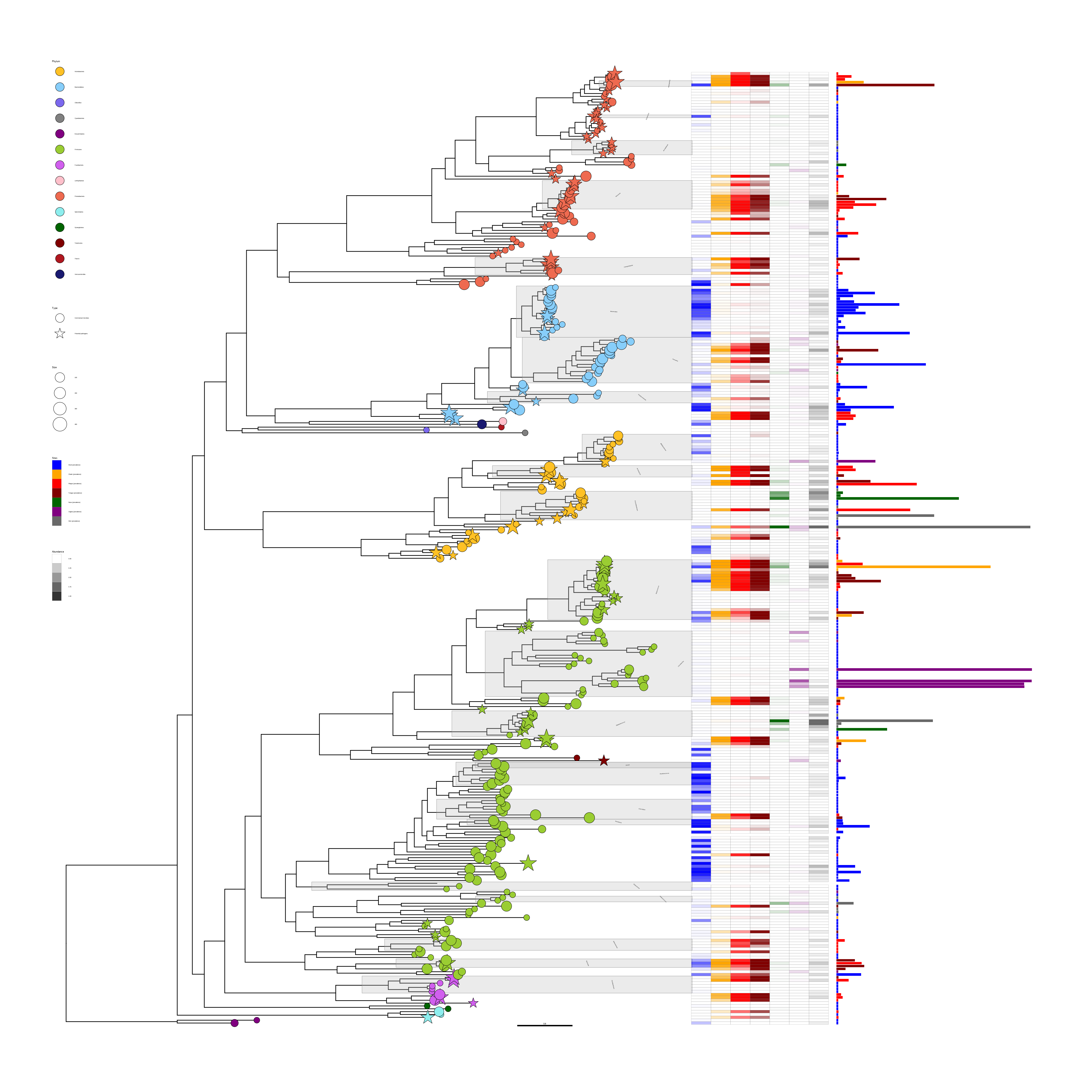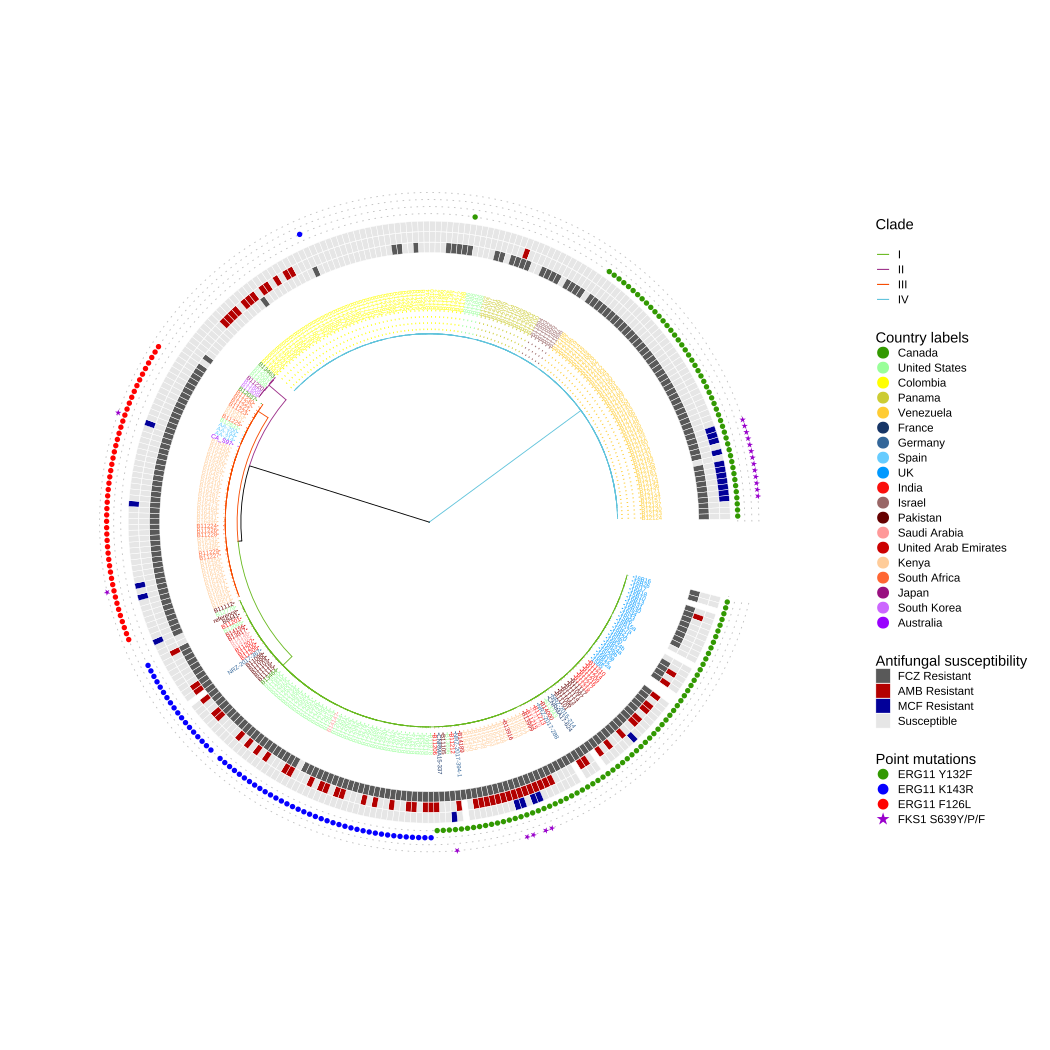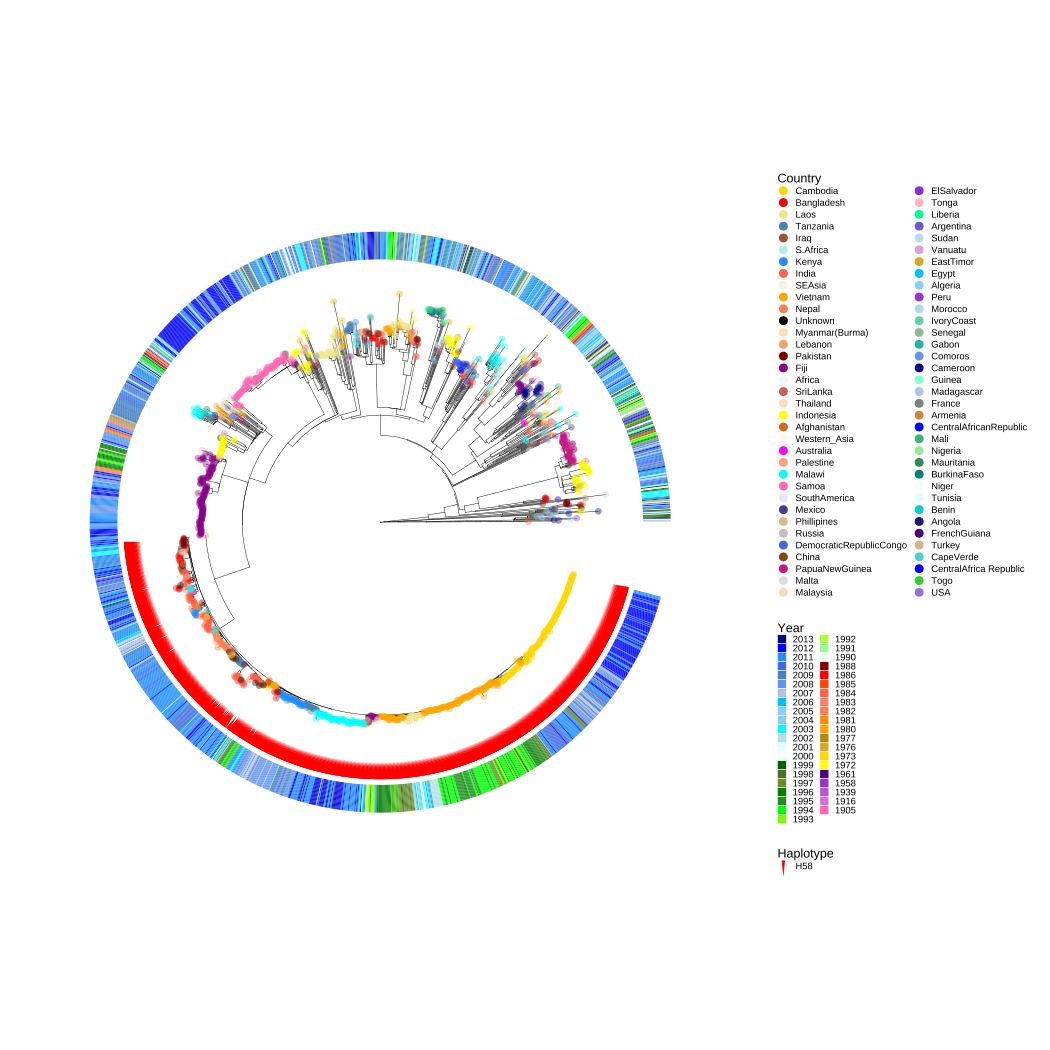# 10 ggtreeExtra for presenting data on circular layout

## 10.1 Introduction

The ggtree package provides programmable visualization and annotation of phylogenetic trees and other tree-like structures. It supports visualizing tree data in multiple layers or with the tree side by side (see also Chapter 7 and ). Although ggtree supports many layouts, the geom_facet() layer only works with rectangular, roundrect, ellipse and slanted layouts to present tree data on different panels. There is no direct supports in ggtree to present data on the outer rings of tree in circular, fan and radial layouts. To solve this issue, we developed the ggtreeExtra package, which allows users to align associated graph layers in outer rings of circular layout tree. In addition, it also works with rectangular tree layout (Figure 10.3).

## 10.2 Aligning graphs to the tree based on tree structure

The ggtreeExtra package provides a layer function, geom_fruit(), to align graphs with the tree side by side. Similar to the geom_facet() layout described in Chapter 7, geom_fruit() internally re-order the input data based on the tree structure and visualize the data using specified geometric layer function with user provided aesthetic mapping and non-variable setting. The graph will be displayed on the outer ring of the tree.

The geom_fruit() is designed to work with most of geom layers defined in ggplot2 and its extensions. The position of the graph (i.e. on the outer ring) is controlled by the position parameter, which accepts a Positioin object. The default value of the position parameter is ‘auto’ and the geom_fruit() layer will guess and determine (hopefully) a suitable position for the specified geometric layer. That means using position_stackx() for geom_bar(), position_dodgex() for geom_violin() and geom_boxplot(), and position_identityx() for others (e.g. geom_point() and geom_tile() etc.). A geometric layer that has a position parameter should be compatible with geom_fruit(), as it allows using position functions defined in the ggtreeExtra package to adjust output layer position. Besides, the geom_fruit() layer allows setting axis and background grid lines for the current layer using the axis.params and grid.params parameters respectively.

The following example uses microbiome data that provided in the phyloseq package and boxplot is employed to visualize species abundance data. The geom_fruit() layer automatically re-arranges the abundance data according to the circular tree structure and visualizes the data using the specific geom function (i.e. geom_boxplot()). Visualizing this dataset using geom_density_ridges() with geom_facet() can be found in Fig. 1 of .

library(ggtreeExtra)
library(ggtree)
library(phyloseq)
library(dplyr)

data("GlobalPatterns")
GP <- GlobalPatterns
GP <- prune_taxa(taxa_sums(GP) > 600, GP)
sample_data(GP)human <- get_variable(GP, "SampleType") %in% c("Feces", "Skin") mergedGP <- merge_samples(GP, "SampleType") mergedGP <- rarefy_even_depth(mergedGP,rngseed=394582) mergedGP <- tax_glom(mergedGP,"Order") melt_simple <- psmelt(mergedGP) %>% filter(Abundance < 120) %>% select(OTU, val=Abundance) p <- ggtree(mergedGP, layout="fan", open.angle=10) + geom_tippoint(mapping=aes(color=Phylum), size=1.5, show.legend=FALSE) p <- rotate_tree(p, -90) p <- p + geom_fruit( data=melt_simple, geom=geom_boxplot, mapping = aes( y=OTU, x=val, group=label, fill=Phylum, ), size=.2, outlier.size=0.5, outlier.stroke=0.08, outlier.shape=21, axis.params=list( axis = "x", text.size = 1.8, hjust = 1, vjust = 0.5, nbreak = 3, ), grid.params=list() ) p <- p + scale_fill_discrete( name="Phyla", guide=guide_legend(keywidth=0.8, keyheight=0.8, ncol=1) ) + theme( legend.title=element_text(size=9), # The title of legend legend.text=element_text(size=7) # The label text of legend, the sizes should be adjust with dpi. ) pFigure 10.1: Phylogenetic tree with OTU abundance distribution. Species abundance distribution were aligned to the tree and visualized as boxplots. The Phylum information was used to color symbolic points on the tree and also species abundance distributions. ## 10.3 Aligning multiple graphs to the tree for multi-dimensional data We are able to add multiple geom_fruit() layers to a tree and circular layout is indeed more compact and efficient for multi-dimensional data. This example reproduce Fig.2 of . The data is provided by GraPhlAn , which contained the relative abundance of microbiome at different body sites. This example demonstrates the abilities of adding multiple layers (heat map and bar plot) to present different types of data (Figure 10.2). library(ggtreeExtra) library(ggtree) library(treeio) library(tidytree) library(ggstar) library(ggplot2) library(ggnewscale) tree <- read.tree("data/HMP_tree/hmptree.nwk") # the abundance and types of microbes dat1 <- read.csv("data/HMP_tree/tippoint_attr.csv") # the abundance of microbes at different body sites. dat2 <- read.csv("data/HMP_tree/ringheatmap_attr.csv") # the abundance of microbes at the body sites of greatest prevalence. dat3 <- read.csv("data/HMP_tree/barplot_attr.csv") # adjust the order dat2Sites <- factor(dat2$Sites, levels=c("Stool (prevalence)", "Cheek (prevalence)", "Plaque (prevalence)","Tongue (prevalence)", "Nose (prevalence)", "Vagina (prevalence)", "Skin (prevalence)")) dat3$Sites <- factor(dat3$Sites, levels=c("Stool (prevalence)", "Cheek (prevalence)", "Plaque (prevalence)", "Tongue (prevalence)", "Nose (prevalence)", "Vagina (prevalence)", "Skin (prevalence)")) # extract the clade label information. Because some nodes of tree are annotated to genera, # which can be displayed with high light using ggtree. nodeids <- nodeid(tree, tree$node.label[nchar(tree$node.label)>4]) nodedf <- data.frame(node=nodeids) nodelab <- gsub("[\\.0-9]", "", tree$node.label[nchar(tree$node.label)>4]) # The layers of clade and hightlight poslist <- c(1.6, 1.4, 1.6, 0.8, 0.1, 0.25, 1.6, 1.6, 1.2, 0.4, 1.2, 1.8, 0.3, 0.8, 0.4, 0.3, 0.4, 0.4, 0.4, 0.6, 0.3, 0.4, 0.3) labdf <- data.frame(node=nodeids, label=nodelab, pos=poslist) # The circular layout tree. p <- ggtree(tree, layout="fan", size=0.15, open.angle=5) + geom_hilight(data=nodedf, mapping=aes(node=node), extendto=6.8, alpha=0.3, fill="grey", color="grey50", size=0.05) + geom_cladelab(data=labdf, mapping=aes(node=node, label=label, offset.text=pos), hjust=0.5, angle="auto", barsize=NA, horizontal=FALSE, fontsize=1.4, fontface="italic" ) p <- p %<+% dat1 + geom_star( mapping=aes(fill=Phylum, starshape=Type, size=Size), position="identity",starstroke=0.1) + scale_fill_manual(values=c("#FFC125","#87CEFA","#7B68EE","#808080","#800080", "#9ACD32","#D15FEE","#FFC0CB","#EE6A50","#8DEEEE", "#006400","#800000","#B0171F","#191970"), guide=guide_legend(keywidth = 0.5, keyheight = 0.5, order=1, override.aes=list(starshape=15)), na.translate=FALSE)+ scale_starshape_manual(values=c(15, 1), guide=guide_legend(keywidth = 0.5, keyheight = 0.5, order=2), na.translate=FALSE)+ scale_size_continuous(range = c(1, 2.5), guide = guide_legend(keywidth = 0.5, keyheight = 0.5, order=3, override.aes=list(starshape=15))) p <- p + new_scale_fill() + geom_fruit(data=dat2, geom=geom_tile, mapping=aes(y=ID, x=Sites, alpha=Abundance, fill=Sites), color = "grey50", offset = 0.04,size = 0.02)+ scale_alpha_continuous(range=c(0, 1), guide=guide_legend(keywidth = 0.3, keyheight = 0.3, order=5)) + geom_fruit(data=dat3, geom=geom_bar, mapping=aes(y=ID, x=HigherAbundance, fill=Sites), pwidth=0.38, orientation="y", stat="identity", ) + scale_fill_manual(values=c("#0000FF","#FFA500","#FF0000","#800000", "#006400","#800080","#696969"), guide=guide_legend(keywidth = 0.3, keyheight = 0.3, order=4))+ geom_treescale(fontsize=2, linesize=0.3, x=4.9, y=0.1) + theme(legend.position=c(0.93, 0.5), legend.background=element_rect(fill=NA), legend.title=element_text(size=6.5), legend.text=element_text(size=4.5), legend.spacing.y = unit(0.02, "cm"), ) pFigure 10.2: Presenting microbiome data (abundance and location) on phylogenetic tree. The tree was annotated with symbolic points, highlighted clades and clade labels. Two geom_fruit() layers was used to visualize location and abundance information. The shape of the tip points indicates the types of microbes (commensal microbes or potential pathogens). The transparency of the heatmap indicates the abundance of the microbes, and the colours of the heatmap indicate different sites of the human body. The bar plot indicates the relative abundance of the most prevalent species at the body sites. The node labels contain taxonomy information in this example, and the information was used to highlight and label corresponding clades using geom_hilight() and geom_cladelab() respectively. The geom_fruit() layer supports rectangular layout. Users can either add a geom_fruit() layer to a rectangular tree (e.g. ggtree(tree_object) + geom_fruit(...)) or using layout_rectangular() to transform a circular layout tree to a rectangular layout tree as demonstrated in Figure 10.3. p + layout_rectangular() + theme(legend.position=c(.05, .7))Figure 10.3: Illustration of using geom_fruit() in rectangular tree layout. ## 10.4 Examples for population genetics The ggtree and ggtreeExtra packages are designed as general tools and can be applied to many research fields, such as infectious disease epidemiology, metagenome, population genetics, evolutionary biology and ecology. We have introduced examples for metagenome research (Figure 10.1 and Figure 10.2). In this session, we present examples for population genetics by reproducing Fig. 4 of and Fig 1 of . library(ggtree) library(ggtreeExtra) library(ggplot2) library(ggnewscale) library(dplyr) library(tidytree) library(ggstar) dat <- read.csv("data/microreact/Candida_auris/microreact-project-Candidaauris-data.csv") tr <- read.tree("data/microreact/Candida_auris/microreact-project-Candidaauris-tree.nwk") countries <- c("Canada", "United States", "Colombia", "Panama", "Venezuela", "France", "Germany", "Spain", "UK", "India", "Israel", "Pakistan", "Saudi Arabia", "United Arab Emirates", "Kenya", "South Africa", "Japan", "South Korea", "Australia") # For the tip points dat1 <- dat %>% select(c("ID", "COUNTRY", "COUNTRY__colour")) dat1$COUNTRY <- factor(dat1$COUNTRY, levels=countries) COUNTRYcolors <- dat1[match(countries,dat$COUNTRY),"COUNTRY__colour"]

# For the heatmap layer
dat2 <- dat %>% select(c("ID", "FCZ", "AMB", "MCF"))
dat2 <- reshape2::melt(dat2,id="ID", variable.name="Antifungal", value.name="type")
dat2$type <- paste(dat2$Antifungal, dat2$type) dat2$type <- unlist(lapply(dat2$type, function(x)ifelse(grepl("Not_", x), "Susceptible", x))) dat2$Antifungal <- factor(dat2$Antifungal, levels=c("FCZ", "AMB", "MCF")) dat2$type <- factor(dat2$type, levels=c("FCZ Resistant", "AMB Resistant", "MCF Resistant", "Susceptible")) # For the points layer dat3 <- dat %>% select(c("ID", "ERG11", "FKS1")) %>% reshape2::melt(id="ID", variable.name="point", value.name="mutation") dat3$mutation <- paste(dat3$point, dat3$mutation)
dat3$mutation <- unlist(lapply(dat3$mutation, function(x)ifelse(grepl("WT",x), NA,x)))
dat3$mutation <- factor(dat3$mutation, levels=c("ERG11 Y132F", "ERG11 K143R",
"ERG11 F126L", "FKS1 S639Y/P/F"))

dat4 <- dat %>% select(c("ID", "CLADE"))
dat4 <- aggregate(.~CLADE, dat4, FUN=paste, collapse=",")
clades <- lapply(dat4$ID, function(x){unlist(strsplit(x,split=","))}) names(clades) <- dat4$CLADE

p <- ggtree(tr=tr, layout="fan", open.angle=15, size=0.2, aes(colour=Clade)) +
scale_colour_manual(
values=c("black","#69B920","#9C2E88","#F74B00","#60C3DB"),
labels=c("","I", "II", "III", "IV"),
guide=guide_legend(keywidth=0.5,
keyheight=0.5,
order=1,
override.aes=list(linetype=c("0"=NA,
)
)
)
) +
new_scale_colour()

p1 <- p %<+% dat1 +
geom_tippoint(aes(colour=COUNTRY),
alpha=0) +
geom_tiplab(aes(colour=COUNTRY),
align=TRUE,
linetype=3,
size=1,
linesize=0.2,
show.legend=FALSE
) +
scale_colour_manual(
name="Country labels",
values=COUNTRYcolors,
guide=guide_legend(keywidth=0.5,
keyheight=0.5,
order=2,
override.aes=list(size=2,alpha=1))
)

p2 <- p1 +
geom_fruit(
data=dat2,
geom=geom_tile,
mapping=aes(x=Antifungal, y=ID, fill=type),
width=0.1,
color="white",
pwidth=0.1,
offset=0.15
) +
scale_fill_manual(
name="Antifungal susceptibility",
values=c("#595959", "#B30000", "#020099", "#E6E6E6"),
na.translate=FALSE,
guide=guide_legend(keywidth=0.5,
keyheight=0.5,
order=3
)
) +
new_scale_fill()

p3 <- p2 +
geom_fruit(
data=dat3,
geom=geom_star,
mapping=aes(x=mutation, y=ID, fill=mutation, starshape=point),
size=1,
starstroke=0,
pwidth=0.1,
inherit.aes = FALSE,
grid.params=list(
linetype=3,
size=0.2
)

) +
scale_fill_manual(
name="Point mutations",
values=c("#329901", "#0600FF", "#FF0100", "#9900CC"),
guide=guide_legend(keywidth=0.5, keyheight=0.5, order=4,
override.aes=list(starshape=c("ERG11 Y132F"=15,
"ERG11 K143R"=15,
"ERG11 F126L"=15,
"FKS1 S639Y/P/F"=1),
size=2)
),
na.translate=FALSE,
) +
scale_starshape_manual(
values=c(15, 1),
guide="none"
) +
theme(
legend.background=element_rect(fill=NA),
legend.title=element_text(size=7), # The size should be adjusted with different devout.
legend.text=element_text(size=5.5),
legend.spacing.y = unit(0.02, "cm")
)
p3Figure 10.4: Antifungal susceptibility and point mutations in drug targets in Candida auris .

In this example, the phylogenetic tree is annotated with different colours to display different clades. The external heatmaps presents the susceptibility to fluconazole (FCZ), amphotericin B (AMB) and micafungin (MCF). The external points display the point mutations in lanosterol 14-alpha-demethylase ERG11 (Y132F, K143R, and F126L) and beta-1,3-D-glucan synthase FKS1 (S639Y/P/F) associated with resistance .

library(ggtreeExtra)
library(ggtree)
library(ggplot2)
library(ggnewscale)
library(treeio)
library(tidytree)
library(dplyr)
library(ggstar)

select(c("id", "country", "country__colour", "year", "year__colour", "haplotype"))
metadata$haplotype <- unlist(lapply(metadata$haplotype, function(x)ifelse(nchar(x)>0,x,NA)))

select(c("country", "country__colour")) %>%
distinct()

select(c("year", "year__colour")) %>%
distinct()
yearcolors <- yearcolors[order(yearcolors$year, decreasing=TRUE),] metadata$country <- factor(metadata$country, levels=countrycolors$country)
metadata$year <- factor(metadata$year, levels=yearcolors$year) p <- ggtree(tr, layout="fan", open.angle=15, size=0.1) p <- p %<+% metadata p1 <-p + geom_tippoint( mapping=aes(colour=country), size=1.5, stroke=0, alpha=0.4 ) + scale_colour_manual( name="Country", values=countrycolors$country__colour,
guide=guide_legend(keywidth=0.3,
keyheight=0.3,
ncol=2,
override.aes=list(size=2,alpha=1),
order=1)
) +
theme(
legend.title=element_text(size=5),
legend.text=element_text(size=4),
legend.spacing.y = unit(0.02, "cm")
)

p2 <-p1 +
geom_fruit(
geom=geom_star,
mapping=aes(fill=haplotype),
starshape=26,
color=NA,
size=2,
starstroke=0,
offset=0,
) +
scale_fill_manual(
name="Haplotype",
values=c("red"),
guide=guide_legend(
keywidth=0.3,
keyheight=0.3,
order=3
),
na.translate=FALSE
)

p3 <-p2 +
new_scale_fill() +
geom_fruit(
geom=geom_tile,
mapping=aes(fill=year),
width=0.002,
offset=0.1
) +
scale_fill_manual(
name="Year",
values=yearcolors\$year__colour,
guide=guide_legend(keywidth=0.3, keyheight=0.3, ncol=2, order=2)
) +
theme(
legend.title=element_text(size=6), # The size should be adjusted with the different devout.
legend.text=element_text(size=4.5),
legend.spacing.y = unit(0.02, "cm")
)
p3Figure 10.5: Population structure of the 1,832 S. Typhi isolates.

This is a rooted maximum-likelihood tree of S. Typhi inferred from 22,145 SNPs , the colours of the tip points represent the geographical origin of the isolates, and the red symbolic points indicate the halotype of H58 lineage. The colour of external heatmap indicates the years of isolation .

## 10.5 Summary

Both geom_facet() and geom_fruit() is becoming more powerful to present data with trees as more geometric layers will be implemented by the ggplot2 community.